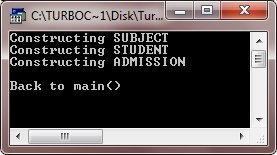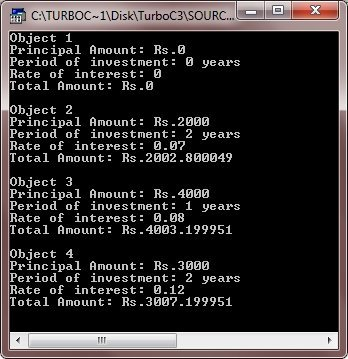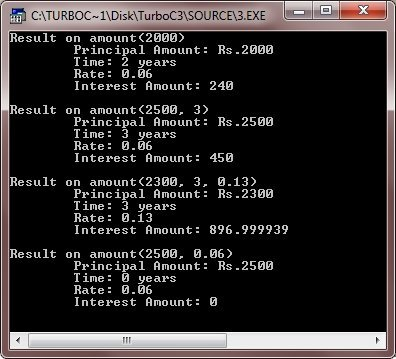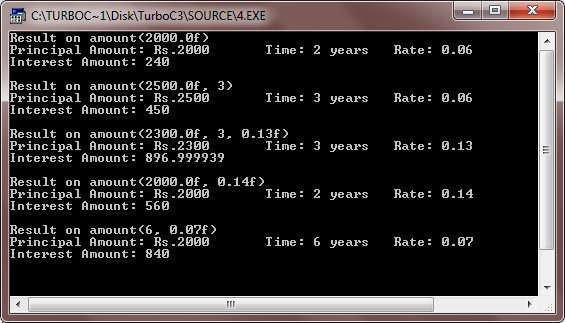# C++ Constructors and Destructors

Constructors and Destructors are special member functions of a class for initializing and disposing of objects belonging to that class.

C++ provides a well defined mechanism, for initializing an object when it is created, by means of a constructors; same way when an object is no more needed, C++ defines a way to scrap it off, by means of a destructors. There can be multiple variations in these. Let's discuss each one of these in details.

## C++ Constructor

A member function with the same name as its class is called as Constructor and it is used to initialize the objects of that class type with a legal initial value.

A constructor is a member function of a class that is automatically called, when an object is created of that class. It (the constructor) has the same name as that of the class's name and its primary job is to initialize the object to a legal initial value for the class.

If a class has a constructor, each object of that class will be initialized before any use is made of the object. Consider the following class having a constructor :

```class Student
{
private :
int rollno;
float marks;
public :
:

/* This ( Below i.e., Student() )is a constructor of class Student.
See it has same name as that of its class and no return type */

Student()
{
rollno = 0;
marks = 0.0;
}
:
};```

See the above class has a member function with the same name as its class has and which provides initial values to its data members. Nowforth, whenever an object of the Student type will be created (declared), the compiler will automatically call the constructor Student::Student() for the newly created object.

Note - Constructors and Destructors have no return type, not even void.

### Need for Constructors

A structure or an array in C++ can be initialized at the time of their declaration. For example, consider the following code fragment :

```struct Student
{
int rollno;
float marks;
};
int main()
{
Student s1 = {0,0.0};
int a = {1,2,3,4,5};
:
}```

But such initialization does not work for a class because the class members have their associated access specifiers. They might not be available to the outside world (outside their class).

There can be an alternative solution to it. If we define a member function (say init()) inside the class to provide initial values, as it shown below :

```class Student
{
private :
int rollno;
float marks;
public :
void init()
{
rollno = 0;
marks = 0.0;
}
:        //other public members
};
int main()
{
Student senior ;          //create an object
senior.init();            //initialize it
:
}```

See, now that one function (called init()) is there to provide initial values, but still the programmer has to explicitly invoke it, in order to initialize the object. In other words, the responsibility of initialization lies solely with the programmer. What if, the programmer fails to invoke init() ? The object, in such case, is full of garbage and might cause havoc in your program.

Then, what is the way out ? Simple, take the responsibility of initialization away from the programmer and give it to the compiler, because it is the compiler that exactly knows when objects are created. Every time an object is created, the compiler will automatically initialize it by invoking the initialization function. And the responsibility is taken over by the compiler, if and only if the initialization function bears the same name as that of the class i.e., a constructor.

Note - A constructor for a class is needed so that the compiler automatically initializes an object as soon as it is created. A class constructor, if defined, is called whenever a program creates an object of that class.

## C++ Constructor Declaration and Definition

A constructor is defined like other member functions of a class. It can be defined either inside the class definition or outside the class definition.

The following code fragment defines a constructor inside the class definition :

```class X
{
int i;
public :
int j, k;
X()
{
i = j = k = 0;    //constructor
}
:                   //other members
};```

This simple constructor (X::X()) is as an inline member function. Constructors can be written as outline functions also as it is shown below :

```class X
{
int i;
public :
int j, k;
X();             //only declaration i.e., prototype here
:                //other members
};
X::X()            //constructor defined outside
{
i = j = k = 0;
}```

In the above given two class definitions, the constructor has been defined as a public member function. You even can define a constructor under private or protected sections. A constructor also, obeys the usual access rules of a class. That means, a private or protected constructor is not available to the non-member functions. In other words, with a private or protected constructor, you cannot create an object of the same class in a non-member function, however, this is allowed in the member functions and friend functions.

### Default Constructors

A constructor that accepts no parameter is called the default constructor. In the previous section, X::X() is the default constructor for class X since it taken no parameter. However, the constructor XYZ :: XYZ() is not a default constructor. With a default constructor, objects are created just the same way as variables of other data types are created. For instance,

`X Ob1;`

will create the object Ob1 of type X by invoking the default constructor. But when the constructor accepts some parameters, the initial values must be passed at the time of object creation.

When a user-defined class does not contains an explicit constructor, the compiler automatically supplies a default constructor, having no arguments. This implicitly-declared default constructor is an inline public members of its class.

For instance, consider the following code snippet :

```class A
{
int i;
public :
void getval(void);
void prnval(void);
:          //member functions definitions
};
A Ob1;       /*uses default constructor for creating Ob1. Since user can use it,
that means, this implicitly defined default constructor is
public member of the class */
Ob1.getval();
Ob1.prnval();```

Having a default constructor simply means that an application can declare instances of the class, since C++ requires that whenever an instance of a class is created, its constructor is called.

Tip - If a class has no explicit constructor defined, the compiler will supply a default constructor.

Note - The default constructor provided by the compiler does not do anything specific. It simply allocates memory to data members of object.

### Parameterized Constructors

A constructor that accepts parameters for its invocation is known as parameterized constructor.

Like other functions you can also write constructors that can accept parameters. Such constructors are called parameterized constructors ( or regular constructors ).

Once you declare a constructor with arguments, the default constructor becomes hidden. After this you cannot invoke the default constructor.

This means that you must always specify the arguments whenever you declare an instance (object) of the class. Consider the following code snippet :

```class Test
{
int ant;
public :
Test(int i)          //constructor with arguments
{
ant = i;
}
:                  //other members
};
int main()
{
Test object1(45);             //ok, Argument value provided
Test object2;                 //Error !! No default constructor available
:
}```

Tip - Declaring a constructor with arguments hides the default constructor.

### Copy Constructor

A copy constructor is a constructor of the form classname (classname &). The compiler will use the copy constructor whenever you initialize an instance using values of another instance of same type. For example,

```Sample S1;         //default constructor used
Sample S2 = S1;        //copy constructor used```

In the above code, for the second statement, the compiler will copy the instance S1 to S2 member by member. If you have not defined a copy constructor, the compiler automatically, creates it and it is public.

You can define your own copy constructor also. A copy constructor takes a reference to an object of the same class an argument. Consider the following class definition :

```class Sample
{
int i, j;
public :
Sample(int a, int b)           //constructor
{
i = a;
j = b;
}
Sample(Sample & s)        //copy constructor
{
j = s.j;
i = s.j;
cout << "\nCopy constructor working \n" ;
}
void print(void)
{
cout << i << j << "\n" ;
}
:
};```

The above definition defines two constructors : one parameterized constructor for when initial values are provided and the other a copy constructor to copy data values of a Sample object onto another. These constructors may be used as follows :

```Sample S1(4, a);        //S1 initialize first constructor used
Sample S2(S1);         //S1 copied to S2. Copy constructor called.
Sample S3 = S1;        //S1 copied to S3. Copy constructor called again.```

But if you given an assignment statement as

`S4 = S1;`

it will not invoke the copy constructor. However, if S4 and S1 are objects of same type, this statement is legal and simply assigns values of S1 to S4, member-by-member.

The process of initializing through a copy constructor is known as copy initialization.

### Order of Constructor Invocation

Here is an example program illustrating order of constructor invocation

```/* C++ Constructors and Destructors - Example Program */

#include<iostream.h>
#include<conio.h>
class SUBJECT
{
int days;
int subjectno;
public:
SUBJECT(int d=123, int sn=101);
void printsubject(void)
{
cout<<"Subject No: "<<subjectno<<"\n";
cout<<"Days: "<<days<<"\n";
}
};
SUBJECT::SUBJECT(int d, int sn)
{
cout<<"Constructing SUBJECT\n";
days=d;
subjectno=sn;
}
class STUDENT
{
int rollno;
float marks;
public:
STUDENT()
{
cout<<"Constructing STUDENT\n";
rollno=0;
marks=0.0;
}
void getvalue(void)
{
cout<<"Enter roll number and marks: ";
cin>>rollno>>marks;
}
void print(void)
{
cout<<"Roll No: "<<rollno<<"\n";
cout<<"Marks: "<<marks<<"\n";
}
};
class ADMISSION
{
SUBJECT sub;
STUDENT stud;
float fees;
public:
ADMISSION()
{
cout<<"Constructing ADMISSION\n";
fees=0.0;
}
void print(void)
{
stud.print();
sub.printsubject();
cout<<"Fees: "<<fees<<"\n";
}
};
void main()
{
clrscr();
ADMISSION adm;
cout<<"\nBack to main()\n";
getch();
}```

Here is the sample run of the above C++ program:### Dynamic Initialization of Objects

The dynamic initialization means that the initial values may be provided during runtime. Even class objects can be initialized dynamically i.e., with the values provided at runtime.

Following code snippet explains it :

```class sample
{
int rollno;
float marks;
public :
sample(int a, float b)
{
rollno = a;
marks = b;
}
sample(sample & s)     //copy constructor
{
rollno = s.rollno;
marks = s.marks;
cout << "\n copy constructor at work \n";
}
:
:                    //other members
};
int main()
{
int x;
float y;
cout << "Enter rollno of the student : ";
cin >> x;
cout << "\n Enter marks of the student : ";
cin >> y;
cout << "\n";
sample s1(x, y);
//dynamic initialization with values x and y
:
}```

The benefit of dynamic initialization is that it provides the flexibility of assigning initial values at run time.

### Constructor Overloading

Just like any other function, the constructor of a class may also be overloaded so that even with different number and types of initial values, an object may still be initialized. For example, consider the following example program (Following program uses an overloaded constructor) :

```/* C++ Constructors and Destructors - Example Program */

#include<iostream.h>
#include<conio.h>
#include<stdlib.h>
class DEPOSIT
{
long int principal;
int time;
float rate;
float totalamount;
public:
DEPOSIT(); 	            	      // #1
DEPOSIT(long p, int t, float r);      // #2
DEPOSIT(long p, int t);               // #3
DEPOSIT(long p, float r);             // #4
void calculateamount(void);
void display(void);
};
DEPOSIT::DEPOSIT()
{
principal = time = rate = 0.0;
}
DEPOSIT::DEPOSIT(long p, int t, float r)
{
principal = p;
time = t;
rate = r;
}
DEPOSIT::DEPOSIT(long p, int t)
{
principal = p;
time = t;
rate = 0.08;
}
DEPOSIT::DEPOSIT(long p, float r)
{
principal = p;
time = 2;
rate = r;
}
void DEPOSIT::calculateamount(void)
{
totalamount = principal + (principal*time*rate)/100;
}
void DEPOSIT::display(void)
{
cout<<"Principal Amount: Rs."<<principal<<"\n";
cout<<"Period of investment: "<<time<<" years\n";
cout<<"Rate of interest: "<<rate<<"\n";
cout<<"Total Amount: Rs."<<totalamount<<"\n";
}
void main()
{
clrscr();
DEPOSIT d1;
DEPOSIT d2(2000, 2, 0.07f);
DEPOSIT d3(4000, 1);
DEPOSIT d4(3000, 0.12f);
d1.calculateamount();
d2.calculateamount();
d3.calculateamount();
d4.calculateamount();
cout<<"Object 1\n";
d1.display();
cout<<"\nObject 2\n";
d2.display();
cout<<"\nObject 3\n";
d3.display();
cout<<"\nObject 4\n";
d4.display();
getch();
}```

Above C++ program will display the following output :The above program constructs different objects by calling different constructors. Each class has exactly one destructor i.e., to a destructor function can not be overloaded.

Here is an another example program illustrates the working of default arguments

```/* C++ Constructors and Destructors - Example Program */

#include<iostream.h>
#include<conio.h>
void amount(float prin, int time=2, float rate=0.06);
void amount(float prin, int time, float rate)
{
cout<<"\tPrincipal Amount: Rs."<<prin<<"\n";
cout<<"\tTime: "<<time<<" years\n";
cout<<"\tRate: "<<rate<<"\n";
cout<<"\tInterest Amount: "<<(prin*rate*time)<<"\n";
}
void main()
{
clrscr();
cout<<"Result on amount(2000)\n";
amount(2000);
cout<<"\nResult on amount(2500, 3)\n";
amount(2500, 3);
cout<<"\nResult on amount(2300, 3, 0.13)\n";
amount(2300, 3, 0.13);
cout<<"\nResult on amount(2500, 0.06)\n";
amount(2500, 0.06);
getch();
}```

Here is the sample output of this C++ program:Here is one more example program illustrates the working of function overloading as compared to default arguments

```/* C++ Constructors and Destructors - Example Program */

#include<iostream.h>
#include<conio.h>
void amount(float prin, int time, float rate)
{
cout<<"Principal Amount: Rs."<<prin;
cout<<"\tTime: "<<time<<" years";
cout<<"\tRate: "<<rate;
cout<<"\nInterest Amount: "<<(prin*time*rate);
}
void amount(float prin, int time)
{
cout<<"Principal Amount: Rs."<<prin;
cout<<"\tTime: "<<time<<" years";
cout<<"\tRate: 0.06";
cout<<"\nInterest Amount: "<<(prin*time*0.06);
}
void amount(float prin, float rate)
{
cout<<"Principal Amount: Rs."<<prin;
cout<<"\tTime: 2 years";
cout<<"\tRate: "<<rate;
cout<<"\nInterest Amount: "<<(prin*2*rate);
}
void amount(int time, float rate)
{
cout<<"Principal Amount: Rs.2000";
cout<<"\tTime: "<<time<<" years";
cout<<"\tRate: "<<rate;
cout<<"\nInterest Amount: "<<(2000*time*rate);
}
void amount(float prin)
{
cout<<"Principal Amount: Rs."<<prin;
cout<<"\tTime: 2 years";
cout<<"\tRate: 0.06";
cout<<"\nInterest Amount: "<<(prin*2*0.06);
}
void main()
{
clrscr();
cout<<"Result on amount(2000.0f)\n";
amount(2000.0f);
cout<<"\n\nResult on amount(2500.0f, 3)\n";
amount(2500.0f, 3);
cout<<"\n\nResult on amount(2300.0f, 3, 0.13f)\n";
amount(2300.0f, 3, 0.13f);
cout<<"\n\nResult on amount(2000.0f, 0.14f)\n";
amount(2000.0f, 0.14f);
cout<<"\n\nResult on amount(6, 0.07f)\n";
amount(6, 0.07f);
getch();
}```

Below is the sample run of the above C++ program:### Special Characteristics of Constructors

The constructor functions have certain special characteristics. These are :

• Constructor functions are invoked automatically when the objects are created.
• If a class has a constructor, each object of that class will be initialized before any use is made of the object.
• Constructor functions obeys the usual access rules. That is, private and protected constructors are available only for member and friend functions, however, public constructors are available for all the functions. Only the functions that have access to the constructor of a class, can create an object of the class.
• No return type (not even void) can be specified for a constructor.
• They cannot be inherited, through a derived class can call the base class constructor.
• A constructor may not be static.
• Default constructors and copy constructors are generated (by the compiler) where needed. Generated constructors are public.
• Like other C++ functions, constructors can also have default arguments.
• It is not possible to take the address of a constructor.
• An object of a class with a constructor cannot be a member of a union.
• Member functions may be called from within a constructor.
• A constructor can be used explicitly to create new objects of its class type, using the syntax.
class-name(expression-list)
For example, Sample obj1 = Sample(13,22.42);

## C++ Destructor

Just as objects are created, so are they destroyed. If a class can have a constructor to set things up, it should also have a destructor to destruct the object. A destructor, as the name itself suggests, is used to destroy the objects that have been created by a constructor. A destructor destroys the values of the object being destroyed.

A destructor is also a member function whose name is the same as the class name but is preceded by tilde('~'). For example, the destructor for the class Sample will bear the name ~Sample().

A destructor takes no arguments, and no return types can be specified for it (not even void). It is called automatically by the compiler when an object is destroyed. (A local auto object, local to a block, is destroyed when the block gets over; a global or static object is destroyed when the program terminates). A destructor cleans up the storage (memory area of the object) that is no longer accessible.

Notice the name of a destructor, it is ~classname(). The symbol ~ refers to a NOT i.e., a destructor is a NOT constructor. A constructor initializes an object and a fragment gives an example of a destructor :

```class Sample
{
int i, j;
public :
Sample(int a, int b)       //constructor
{
i = a;
j = b;
}
~Sample()
{
cout << "Destructor at work\n" ;
}
:                  //other members
};
int main()
{
Sample s1(3, 4);        //local object s1 constructed with values 3 & 4 using Sample()
.
.
.
/* automatically s1 is destructed at the end of the block using destructor ~Sample()
}```

### Need for Destructors

During construction of an object by the constructor, resources may be allocated for use. For example, a constructor may have opened a file and a memory area may be allotted to it. Similarly, a constructor may have allocated memory to some other objects.

These allocated resources must be deallocated before the object is destroyed. Now whose responsibility is it ? A destructor volunteers here for this task and performs all clean-up tasks (like closing a file, deallocating and releasing memory area) automatically. Therefore, a destructor is equally useful as a constructor is.

## C++ Destructor Declaration and Definition

A destructor is a member function and can be defined like any other member function. However, it does not take any argument neither does it return any values.

A destructor being a member function obeys the usual access rules for a member functions. That is, if defined as a private or protected member function, it becomes available only for member functions and only these can create, use, and destroy objects of this class type. However, if a class defines a public destructor, its objects can be created, used, and destroyed by any function. Following code snippet illustrates it :

```class Sample
{
int i, j;
~Sample()                  //private destructor.
{
cout << "Destructing\n" ;
}
public :
Sample()            //constructor.
{
i = 0;
j = 0;
}
void memb1(void);
void memb2(void);
};
void Sample::memb1(void)
{
Sample s1;        /*valid. object can be used here. It is a member function and
thus has an access to its private destructor */
}
void Sample::memb2(void)
{
Sample s2;       /*valid. abc() is a friend function and thus has an access to
sample's private members. */
.
.
.
}
int main()
{
Sample s4;         /* invalid main() is a non-member function and thus
cannot access private members (destructor also as it is private). */
.
.
.
}```

If you fail to define a destructor for a class, the compiler automatically generates one, the default destructor. If more than one object is being destructed, the destructors are invoked in the reverse order in which the constructors were called.

For example,

```{
Sample s1;                     // s1 constructed
Sample s2;                     // s2 constructed
Sample s3;	       // s3 constructed
:
// s3 destructed
// s4 destructed
}					// s5 destructed```

Note - Generally, a destructor should be defined under the public section of a class, so that its objects can be (used and then) destroyed in any function.

### Some Characteristics of Destructors

The destructors have some special characteristics associated. These are :

• Destructor functions are invoked automatically when the objects are destroyed.
• You can have only one destructor for a class. In other words, destructors can't be overloaded.
• If a class has a destructor, each object of that class will be de-initialized before the object goes out of scope. (Local objects at the end of the block defining them and global and static objects at the end of the program.)
• Destructor functions also, obey the usual access rules as other member functions do.
• No argument can be provided to a destructor, neither does it return any value.
• They cannot be inherited.
• A destructor may not be static.
• It is not possible to take the address of a destructor.
• Member functions may be called from within a destructor.
• An object of a class with a destructor cannot be a member of a union.

Tools
Calculator

Quick Links
Signup - Login - Give Online Test